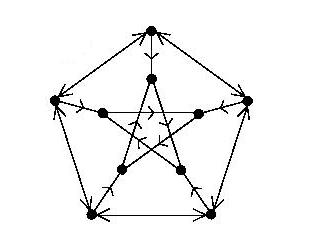# Guide for creating/editing content

### Step by step instructions

Field Guidelines
Title The title used by the Garden for reference purposes.
Statement A concise statement of the conjecture. Please keep this short, even at the cost of postponing essential definitions to the body.
Importance
 Outstanding ✭✭✭✭ One of the top problems in the area. High ✭✭✭ A problem which has received attention in the literature. Medium ✭✭ A problem which the author considers worthy of publication. Low ✭ A problem which either appears of low value, or has not been seriously considered.
Authors The authors of the conjecture in a list separated by semicolons. Please use the format Lastname, Firstname I., and please pause to let autocomplete "guess" author names to prevent multiple copies of the same author.
Area » Topic » Subtopic The subject the open problem belongs in. Every problem must be assigned an Area, but problems need not be assigned a Topic or Subtopic. Please assign your problem the most reasonable classification possible, and contact us ("Contact" button in upper right hand corner) if the appropriate subject does not exist.
Keywords key words used for reference purposes. Separate by semicolons.
Recommended for undergrads "yes" if the problem looks suitable for an undergraduate and "no" otherwise.
Prize If there is a prize for this conjecture, a description of it, and who offered it.
Status "open" if the problem is unsolved, and "solved" otherwise. If the problem is solved, indicate the solver and reference for the paper in the extra field.
Discussion A discussion of the problem. This is a LaTeX field where you can use all of the commands in the table below. We encourage you to use Wikipedia for definitions of standard terms. If Wikipedia is lacking here, update it!
Related Problems A list of related problems.
Bibliography A bibliography for the problem. We indicate original appearances of the problem with an *, and provide links to the paper and to MathSciNet when possible.

### Supported LaTeX

 Command Appearanceemph{italics} italicstextbf{bold} bold $e^{i \pi}in {\mathbb Z}$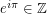$$e^{i \pi}in {\mathbb Z}$$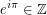begin{problem} $P = NP?$end{problem} (also works for conjecture, question, theorem, proposition, observation, statement, lemma) Problem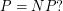begin{enumerate}item lineitem planeend{enumerate} \item line \item planebegin{itemize}item triangleitem squareend{itemize} \item triangle \item squarebegin{proof} induction on $n$.end{proof} Proof   induction on.begin{definition} $zeta(s) =sum_{n=1}^{infty}frac{1}{n^s}$end{definition} Definition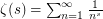Note: LaTeX includes the files amsmath, amsfonts, amssymb, latexsym, and mathrsfs.

 Link to Command Appearance WikipediaDef[graph]{graph theory} graph (links to the Wikipedia page for graph theory) Open problem in the Garden (by number)OPrefnum[5-Flow Conjecture]{126} 5-Flow Conjecture (links to the open problem with node 126*). Open problem in the Garden (by name)OPref[5-Flow Conjecture]{5_flow_conjecture} 5-Flow Conjecture (links to the open problem whose url ends with 5_flow_conjecture). General external linkhref[link to google]{http://www.google.com} link to google (links to http://www.google.com) The ArXivarxiv[The strong perfect graph theorem]{math.CO/0212070} The strong perfect graph theorem (links to the ArXiv paper math.CO/0212070) MathSciNetMRhref{0579077} MathSciNet (links to the MathSciNet Review MR0579077)
• Then you can useImage{file name} orImage[alternative text]{file name}
Example:Image[A bidirected orientation of the Petersen graph]{bi_pet.jpg} yields